Function Repository Resource:

# CircleProperties

Get an Association of the properties of a given circle

Contributed by: Wolfram|Alpha Math Team
 ResourceFunction["CircleProperties"][expr,{x,y}] returns an Association of properties of the circle defined by expr in terms of the variables x and y.

## Details and Options

The input expr can be an equation or an Association of circle properties. In this case, ResourceFunction["CircleProperties"] will calculate and return the full set of properties.
Circle properties include "Equation", "Radius", "Circumference", "Center" and "AreaEnclosed".
If the given expr describes a conic section other than a circle, ResourceFunction["CircleProperties"] will issue a message and return an Association of properties pertaining to the correct conic section.

## Examples

### Basic Examples (1)

Compute the properties of a circle:

 In:=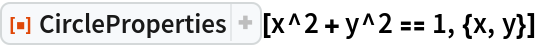Out=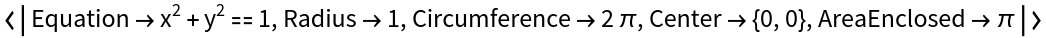### Scope (2)

Compute the full set of properties of a circle described using a subset of properties:

 In:=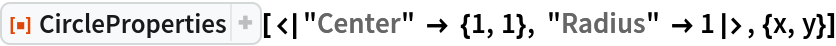Out=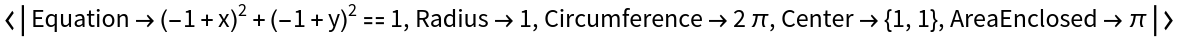Compute the properties of a general circle centered at {h,k} with radius r:

 In:=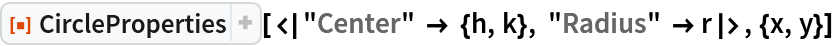Out=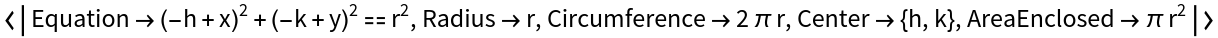### Properties and Relations (1)

Use the resource function InteractiveConicPlot to visualize the results of CircleProperties:

 In:=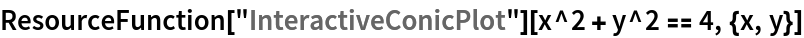Out=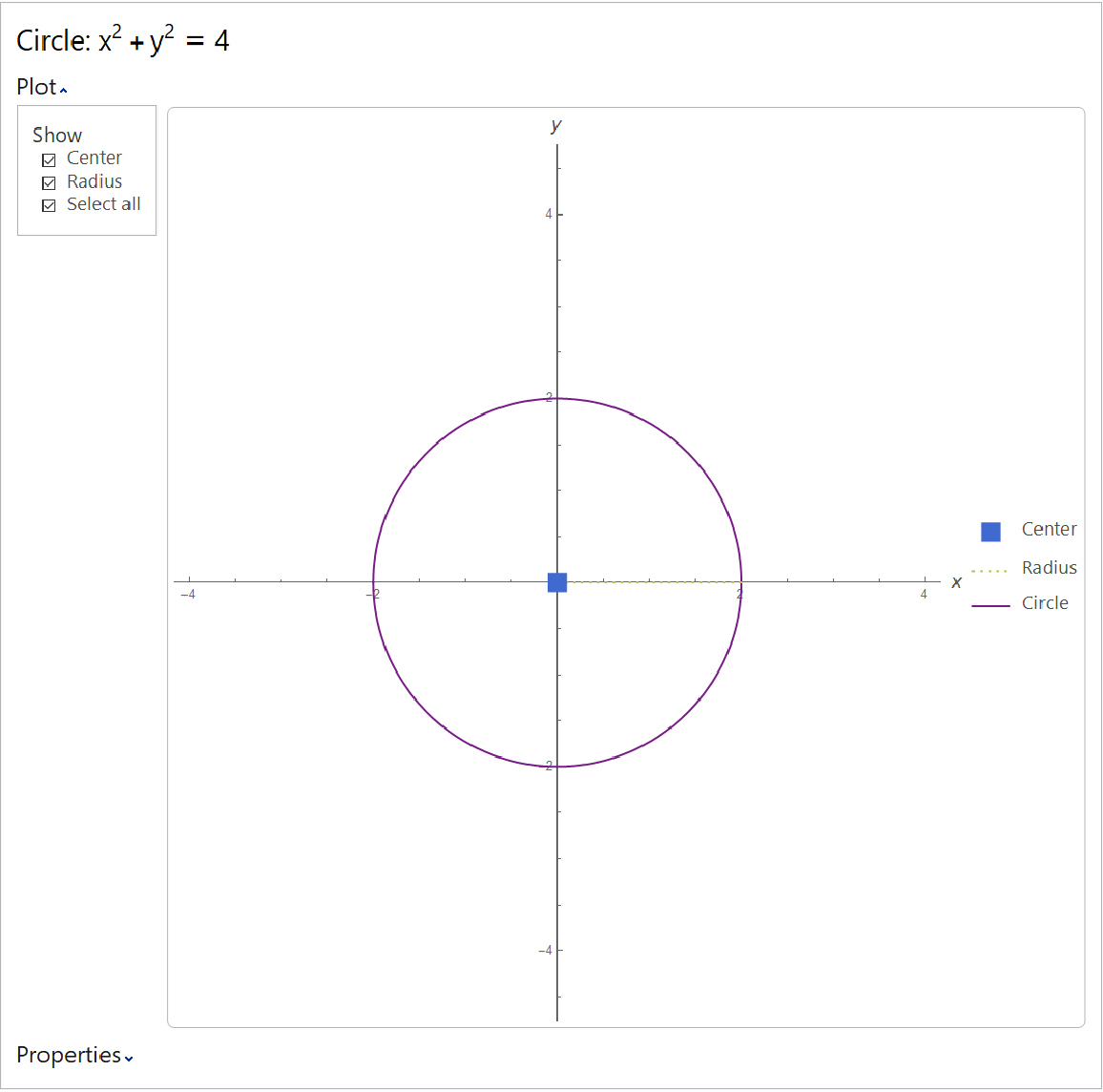### Possible Issues (1)

If the input equation or Association does not describe a circle but describes another conic section, CircleProperties will return an Association of properties pertaining to the correct conic section, including its "Type" ("Parabola", "Hyperbola" or "Ellipse"):

 In:=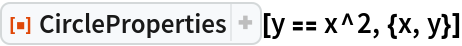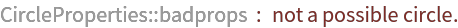Out=## Publisher

Wolfram|Alpha Math Team

## Version History

• 2.0.0 – 23 March 2023
• 1.0.0 – 01 May 2020